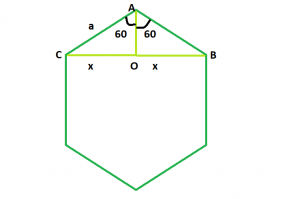# Diagonal of a Regular Hexagon

Given an integer a which is the side of a regular hexagon, the task is to find and print the length of its diagonal.

Examples:

Input: a = 6
Output: 10.38

Input: a = 9
Output: 15.57

## Recommended: Please try your approach on {IDE} first, before moving on to the solution.Approach: We know that the sum of interior angles of a polygon = (n – 2) * 180 where, n is the number of sides of the polygon.
So, sum of interior angles of a hexagon = 4 * 180 = 720 and each interior angle will be 120.
Now, we have to find BC = 2 * x. If we draw a perpendicular AO on BC, we will see that the perpendicular bisects BC in BO and OC, as triangles AOB and AOC are congruent to each other.
So, in triangle AOB, sin(60) = x / a i.e. x = 0.866 * a
Therefore, diagonal length will be 2 * x i.e. 1.73 * a.

Below is the implementation of the above approach:

## C++

 `// C++ Program to find the diagonal ` `// of a regular hexagon ` `#include ` `using` `namespace` `std; ` ` `  `// Function to find the diagonal ` `// of a regular hexagon ` `float` `hexDiagonal(``float` `a) ` `{ ` ` `  `    ``// Side cannot be negative ` `    ``if` `(a < 0) ` `        ``return` `-1; ` ` `  `    ``// Length of the diagonal ` `    ``float` `d = 1.73 * a; ` `    ``return` `d; ` `} ` ` `  `// Driver code ` `int` `main() ` `{ ` `    ``float` `a = 9; ` `    ``cout << hexDiagonal(a) << endl; ` `    ``return` `0; ` `} `

## Java

 `// Java Program to find the diagonal  ` `// of a regular hexagon ` ` `  `public` `class` `GFG ` `{ ` ` `  `    ``// Function to find the diagonal  ` `    ``// of a regular hexagon  ` `    ``static` `double` `hexDiagonal(``float` `a)  ` `    ``{  ` `     `  `        ``// Side cannot be negative  ` `        ``if` `(a < ``0``)  ` `            ``return` `-``1``;  ` `     `  `        ``// Length of the diagonal  ` `        ``double` `d = (``double``)``1.73` `* a;  ` `        ``return` `d;  ` `    ``}  ` `     `  `    ``// Driver code  ` `    ``public` `static` `void` `main(String []args) ` `    ``{  ` `        ``float` `a = ``9``;  ` `        ``System.out.println(hexDiagonal(a)) ;  ` `    ``}  ` `    ``// This code is contributed by Ryuga ` `} `

## Python3

 `# Python3 Program to find the diagonal ` `# of a regular hexagon ` ` `  `# Function to find the diagonal ` `# of a regular hexagon ` `def` `hexDiagonal(a): ` ` `  `    ``# Side cannot be negative ` `    ``if` `(a < ``0``): ` `        ``return` `-``1``; ` ` `  `    ``# Length of the diagonal ` `    ``d ``=` `1.73` `*` `a; ` `    ``return` `d; ` ` `  ` `  `# Driver code ` `a ``=` `9``; ` `print``(hexDiagonal(a)); ` ` `  `# This code is contributed ` `# by Akanksha Rai `

## C#

 `// C# Program to find the diagonal   ` `// of a regular hexagon  ` `using` `System ;  ` `public` `class` `GFG  ` `{  ` `   `  `    ``// Function to find the diagonal   ` `    ``// of a regular hexagon   ` `    ``static` `double` `hexDiagonal(``float` `a)   ` `    ``{   ` `       `  `        ``// Side cannot be negative   ` `        ``if` `(a < 0)   ` `            ``return` `-1;   ` `       `  `        ``// Length of the diagonal   ` `        ``double` `d = (``double``)1.73 * a;   ` `        ``return` `d;   ` `    ``}   ` `       `  `    ``// Driver code   ` `    ``public` `static` `void` `Main()  ` `    ``{   ` `        ``float` `a = 9;   ` `        ``Console.WriteLine(hexDiagonal(a)) ;   ` `    ``}   ` `    ``// This code is contributed by Subhadeep ` `}  `

## PHP

 ` `

Output:

```15.57
```

Attention reader! Don’t stop learning now. Get hold of all the important DSA concepts with the DSA Self Paced Course at a student-friendly price and become industry ready.

My Personal Notes arrow_drop_upCheck out this Author's contributed articles.

If you like GeeksforGeeks and would like to contribute, you can also write an article using contribute.geeksforgeeks.org or mail your article to contribute@geeksforgeeks.org. See your article appearing on the GeeksforGeeks main page and help other Geeks.

Please Improve this article if you find anything incorrect by clicking on the "Improve Article" button below.